# The Element's-Eye View

There are three things to keep in mind when it comes to set-theory proofs (which most of our proofs hereafter will be):

1. Sets are defined by their membership. Because of this, we nearly always take an element's-eye view of any proof involving sets. Notice that in the proofs we presented above, the first thing we did to get going was say something like Let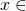. . .".
2. Generally, the way to go to prove two sets are equal is to show (separately) that each is a subset of the other.
3. Keep in mind what your goal is. That is, pay attention to the consequent. Your goal is to translate from the language of the consequent into the language of the antecedent.

These three principles are not only good advice for writing a proof, but they are in fact good advice for {\em figuring out} a proof as well. The following examples will illustrate principles 1 and 3.

Theorem. If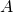and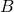are sets with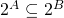, then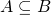.

Proof. Letandbe set with. Since we have to show, let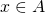. Then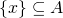, so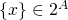. Since, we have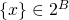. But this means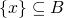. Since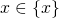, we see that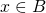.

So we've shown everyalso has, i.e..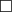Theorem. Ifandare sets with, then.

Proof. Letandbe sets with. Since we have to show, let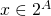. Then. Since, and set inclusion is transitive, we have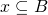. But this is exactly the statement that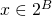.

So we've show that everyalso has, i.e..Notice that in both cases, we were concerned with the {\em consequent} of the conditional we'd be asked to show (principle III), and that we proceeded by following elements (principle I). In the first proof, we translated from the language of elements ofandto the language of subsets ofandto the language of elements of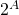and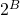, applied our assumption, and translated back. In the second proof, we translated from the language of elements ofandto the language of subsets ofand, applied our assumptions, and translated back.

Activity Consider the following couplet:

Heffalumps and woozles are very confuzle.
A heffalump or woozle's very sly.

Explain why both lines are talking about the same thing as follows. First, give a mathematical name to what they describe. Then explain why each line takes a different perspective on that mathematical object, and why those perspectives are not contradictory, the difference between and" and or" notwithstanding.

Published in sets.